• English
• Account
• API
• Integrations
• Enterprise
• Resources
Using logical operators to compare field values
• 05 Jul 2022
• Dark
Light

# Using logical operators to compare field values

• Dark
Light

Article Summary

Logical operators allow you to compare values in one field to another (or multiple fields to multiple others!). See the operators listed below for an introduction to their symbols and usage.

## Operators

Operator

Symbol

Use

Example

Greater than

`>`

Compare if one number is greater than another

`IF(5 > 5 ...`

Less than

`<`

Compare if one number is less than another

`IF(5 < 5 ...`

Greater than or equal to

`>=`

Compare if one number is greater than or equal to another

`IF({Field A} >= {Field B} ...`

Less than or equal to

`<=`

Compare if one number is less than or equal to another

`IF({Field A} <= {Field B} ...`

Equal to

`=`

Compare if one value is equal to another value

`5 = 5`

Does not equal

`!=`

Check if one value is not equal to another value

`IF({Field A} != {Field B} ...`

## Greater than or less than

For the following examples, assume that you want to compare income against expenses on a weekly basis. We'll run a few different calculations to see how to compare these two numbers.

## Greater than

First, check if income is greater than expenses. If income is greater than expenses, the result of the formula will be a 1 (meaning true ), and if not, a 0 (meaning false ).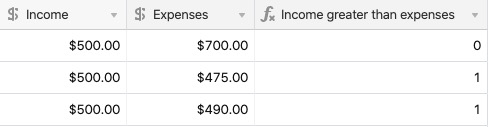`{Income} > {Expenses}`

## Less than

Now, check if income is less than expenses. If income is less than expenses, the result of the formula will be a 1, and if not, a 0.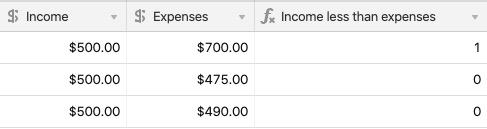`{Income} < {Expenses}`

## Greater than or equal to

Similar to the formulas above, we can compare if income is greater than or equal to expenses (still returning a 1 or 0). The difference is most obvious in the below table when the Income and Expenses are equal—the formula returns 1.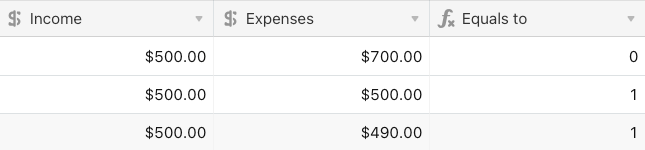`{Income} >= {Expenses}`

## Less than or equal to

Now we can check the opposite, let's compare if income is less than or equal to expenses by changing the operator.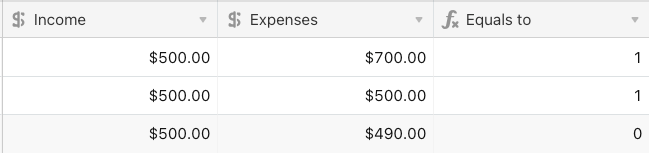`{Income} <= {Expenses}`

## Equal

Check if income is exactly equal to expenses.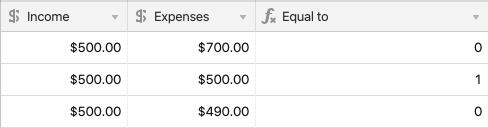`{Income} = {Expenses}`

## Does not equal

Likewise, we can check if a value is not equal to another by adding a ! before the = .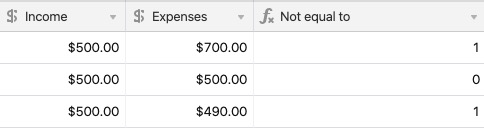`{Income} != {Expenses}`